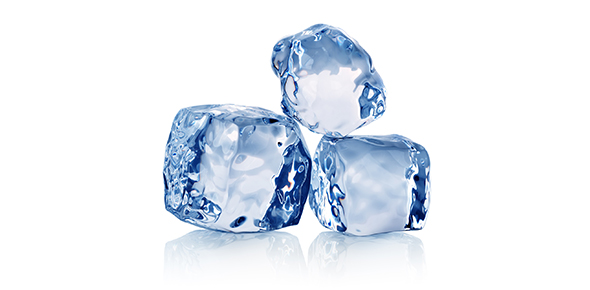# Characteristics Of Solids MCQ Quiz!

13 Questions | Attempts: 737
ShareSettings• 1.
The temperature at which two or more than two types of crystals exist in equilibrium is called?
• A.

Melting point

• B.

Transition temperature

• C.

Eutectic temperature

• D.

Boiling point

• 2.
The solid in which atoms, ions, or molecules have random non-repetitive three dimensional arrangements are termed as:
• A.

Crystal

• B.

Glasses

• C.

Alloys

• D.

Amalgams

• 3.
Crystal lattice is the arrangement of particles in:
• A.

One dimension

• B.

Two dimensions

• C.

Three dimensions

• D.

Four dimensions

• 4.
The nature of bond in diamond is:
• A.

Ionic

• B.

Covalent

• C.

Molecular

• D.

Metallic

• 5.
Lattice energy is also called:
• A.

Crystal energy

• B.

Ionization energy

• C.

Energy of affinity

• D.

Bond energy

• 6.
When gaseous ions are allowed to form a crystal, energy:
• A.

Is evolved

• B.

Is absorbed

• C.

Is sometimes absorbed and sometimes released

• D.

Has no effect

• 7.
NaF and MgO have both atomic ratio 1:1 in their crystals such property is:
• A.

Polymorphism

• B.

Isomorphism

• C.

Isotropy

• D.

Allotropy

• 8.
Electric current can pass through graphite in one direction but not through other directions of crystal such property is called:
• A.

Allotropy

• B.

Anisotropy

• C.

Isomorphism

• D.

Polymorphism

• 9.
Iron acts as an electrical conductor due to:
• A.

Electron cloud

• B.

Free protons

• C.

Free neutrons

• D.

Free ions

• 10.
What method could be used to obtain copper from mixture of powdered copper and sodium chloride:
• A.

Heating the mixture

• B.

Fractional distillation of the mixture

• C.

Passing an electric current through the mixture

• D.

Adding excess water to the mixture and filtering

• 11.
Lead and zinc can be separated by distillation because they have different:
• A.

Boiling point

• B.

Densities

• C.

Mass numbers

• D.

Reactivities

• 12.
An insoluble solid was dropped into a measuring cylinder containing 50 cm3 of water. What will be the effect on volume of water?
• A.

15 cm3

• B.

17 cm3

• C.

50 cm3

• D.

65 cm3

• 13.
The spontaneous mixing of particles is called:
• A.

Evaporation

• B.

Sublimation

• C.

Diffusion

• D.

Boiling

## Related TopicsBack to top
×

Wait!
Here's an interesting quiz for you.